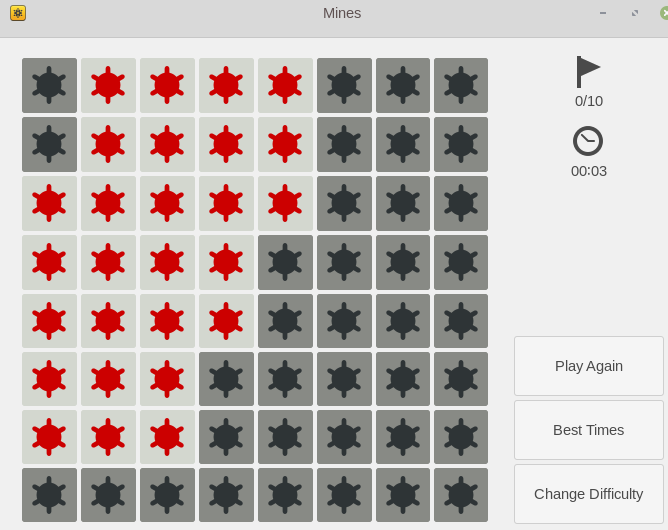Just an update regarding my last post. I was asked to write a code to change other’s process memory and it was straightforward as well.

``````    --- /home/ziviani/temp/mines.cpp.1
+++ /home/ziviani/temp/mines.cpp
@@ -77,7 +77,7 @@
}

string mem_path("/proc/" + string(argv) + "/mem");
-    int fd = open(mem_path.c_str(), O_RDONLY);
+    int fd = open(mem_path.c_str(), O_RDWR);
if (fd < 0) {
cerr << "cannot open " << mem_path << ".\n";
return 3;
@@ -93,6 +93,7 @@

+    int32_t test = 1;
for (int i = 0; i < frame.height; ++i) {
for (int j = 0; j < frame.width; ++j) {
auto index = 8 * (mine_pos * j + i);
@@ -100,10 +101,12 @@
static_cast<off_t>((location + index)));
static_cast<off_t>(has_mine_p + 0x20));
+            pwrite(fd, &test, sizeof(int32_t), static_cast<off_t>(has_mine_p + 0x20));
cout << has_mine << " ";
}
cout << endl;
}
+
close(fd);

return 0;
``````

If you don’t see what I did, I set 1 to the whole map. In other words, mines in every cell. :-)